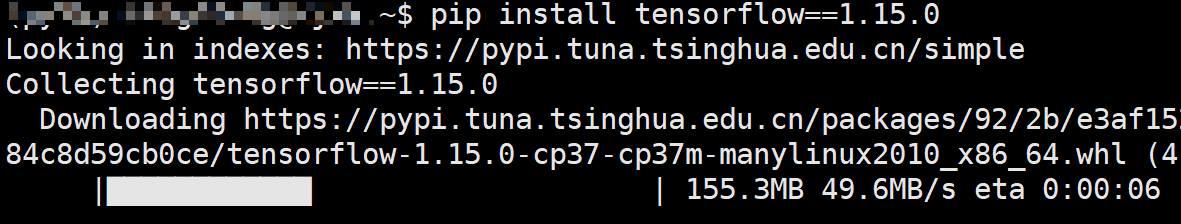# Tensorflow实现线性回归

## 2 框架介绍

### 2.0 安装 tensorflow

pip install tensorflow==1.15.0### 2.1 导入相关包

import tensorflow as tf
from sklearn.preprocessing import StandardScaler
import numpy as np


tensorflow.keras.datasets中内置了一些比较常用的数据集，并且Tensorflow在各个数据集中均实现了load_data这个方法来对数据集进行载入。

## Modules

boston_housing module: Boston housing price regression dataset.

cifar10module: CIFAR10 small images classification dataset.

cifar100module: CIFAR100 small images classification dataset.

fashion_mnist module: Fashion-MNIST dataset.

imdbIMDB sentiment classification dataset.

mnistmodule: MNIST handwritten digits dataset.

reutersmodule: Reuters topic classification dataset.

### 2.2 载入数据

def load_boston_data():
(x_train, y_train), (x_test, y_test) = load_data(test_split=0.3)
ss = StandardScaler()
x_train = ss.fit_transform(x_train)
x_test = ss.transform(x_test)
return x_train, y_train, x_test, y_test


### 2.3 定义正向传播

def forward(x, w, b):
return tf.matmul(x, w) + b


### 2.4 定义损失

def MSE(y_true, y_pred):
return 0.5*tf.reduce_mean(tf.square(y_true - y_pred))


### 2.5 训练模型

def train(x_train, y_train, x_test, y_test):
learning_rate = 0.1
epochs = 300
m, n = x_train.shape
x = tf.placeholder(dtype=tf.float32, shape=[None, n], name='input_x')
y = tf.placeholder(dtype=tf.float32, shape=[None], name='input_y')
w = tf.Variable(tf.truncated_normal(shape=[n, 1],
mean=0, stddev=0.1,
dtype=tf.float32))
b = tf.Variable(tf.constant(0, dtype=tf.float32, shape=))
y_pred = forward(x, w, b)
loss = MSE(y, y_pred)
with tf.Session() as sess:
sess.run(tf.global_variables_initializer())
for epoch in range(epochs):
feed_dict = {x: x_train, y: y_train}
l, _ = sess.run([loss, train_op], feed_dict=feed_dict)
print("[{}/{}]----loss on train:{:.4}".format(epoch, epochs, l))
if epoch % 10 == 0:# 每隔10轮迭代输出一次信息
feed_dict = {x: x_test, y: y_test}# 喂入测试集
l = sess.run(loss, feed_dict=feed_dict)# 计算测试集上的损失
print("[{}/{}]----loss on test:{:.4}-----RMSE: {:.4}".
format(epoch, epochs, l, np.sqrt(l)))


• tf.placeholder

在**上一篇文章**中，我们已经介绍了什么是占位符，但是并没有介绍其用法。在声明一个placeholder时，我们必须要指定其类型dtype，形状shape，以及可选的name。同时，由于在执行计算图的过程中，每个输入的样本数可能不一样，所以shape的第一个维度可以设置为None，例如在训练和测试时batch的大小可能不一样，如果这种情况下设为定值那么就会报错：

ValueError: Cannot feed value of shape (152, 13) for Tensor ‘input_x:0’, which has shape ‘(354, 13)’

另外需要说明的就是参数name，它是一个可选参数。在Tensorflow中，几乎所有tf.打头的类或者方法都有name这么一个参数（例如tf.Variable(),tf.square()等），并且都是可选的。因此初学者就会感到奇怪，这个name到底有什么用，定义变量的时候不是指定了变量名吗？怎么还存在name这么一个参数？这其实就要从Tensorflow的机制说起，Tensorflow在执行计算图时对于各种变量以及op的识别依赖的就是其对应的名称，而我们定义的变量名只是用于用户角度区分。例如上面x=placeholder(...,name='input_x')这个占位符，用户通过x来对其辨识，而Tensorflow内部则是通过input_x来进行辨识。到目前为止我们并没有发现name参数的利用价值，但是当实现一些特殊操作时就会体现（后面会有示例）。

• tf.Variable()

Variable翻译过来就是变量的意思，在Tensorflow中各类网络权重参数都需要通过其来进行定义。同时，Variable需要传入的第一个参数就是初始值，而tf.truncated_normal()就是用来对其进行初始化。

• tf.truncated_normal()

截断正太分布，所谓截断就是对不符合条件（大于平均值两个标准差）的值进行舍弃并重新产生。meanstddev分别表示均值和标准差。tf.constant()则是定义一个常数张量。

• GradientDescentOptimizer()

梯度下降优化器，这里也就是通过梯度下降来对网络的权重进行更新，其至少需要接收一个学习率作为参数。同时，其实例化的minimize()方法需要传入我们要最小化的损失函数。

• tf.Session()

开启一个会话模式，因为后续我们需要通过sess.run()来执行计算图。而global_variables_initializer()则是用于对之前所有定义的Variable()进行初始化赋值操作（声明的时候并没有完成赋值操作）。

• feed_dict
对于前面定义的所有的placeholder，在启动计算图时都需要喂入相应的真实数据。在Tensorflow中，我们将以一个字典的形式把所有占位符需要的东西传进去。注意，字典的key就是占位符的名称，value就是需要传入的值。

• l,_ = run([loss, train_op])

由于我们需要输出查看具体的损失值，所以要将执行loss的计算；同时，我们需要更新网络权重，所以要执行train_op这个优化器操作。最后，我们用l来接收返回的损失值，_来忽略traip_op返回的值。

### 2.6 运行结果

[0/300]----loss on train:294.8
[0/300]----loss on test:250.5-----RMSE: 15.83
[1/300]----loss on train:247.1
[2/300]----loss on train:208.5
[3/300]----loss on train:177.3
[4/300]----loss on train:151.9
[5/300]----loss on train:131.4
[6/300]----loss on train:114.8
[7/300]----loss on train:101.3
[8/300]----loss on train:90.43
[9/300]----loss on train:81.59
[10/300]----loss on train:74.44
[10/300]----loss on test:66.14-----RMSE: 8.133


# 3 总结

### 引用

内置数据集：https://www.tensorflow.org/versions/r1.15/api_docs/python/tf/keras/datasets

### 近期文章

你们要的Tensorflow入坑指南来了

简单谈谈Tensorflow的运行机制09-212642
11-09321309-154416
05-07523
08-112687
02-0834
04-17231
12-0218
09-1263
06-131837